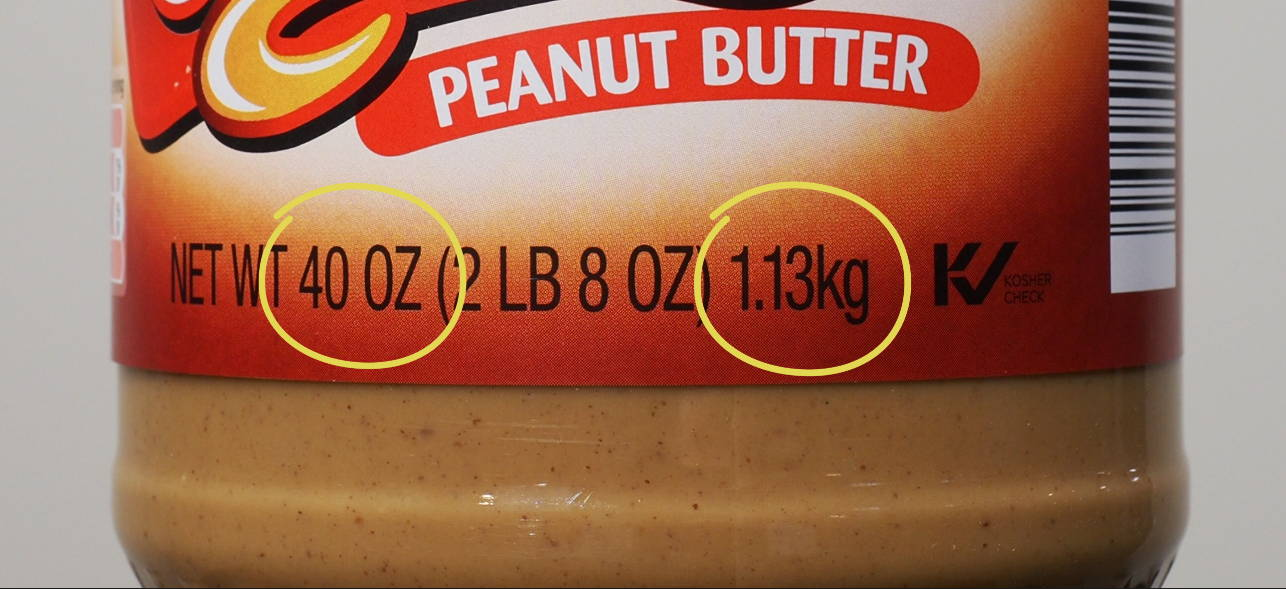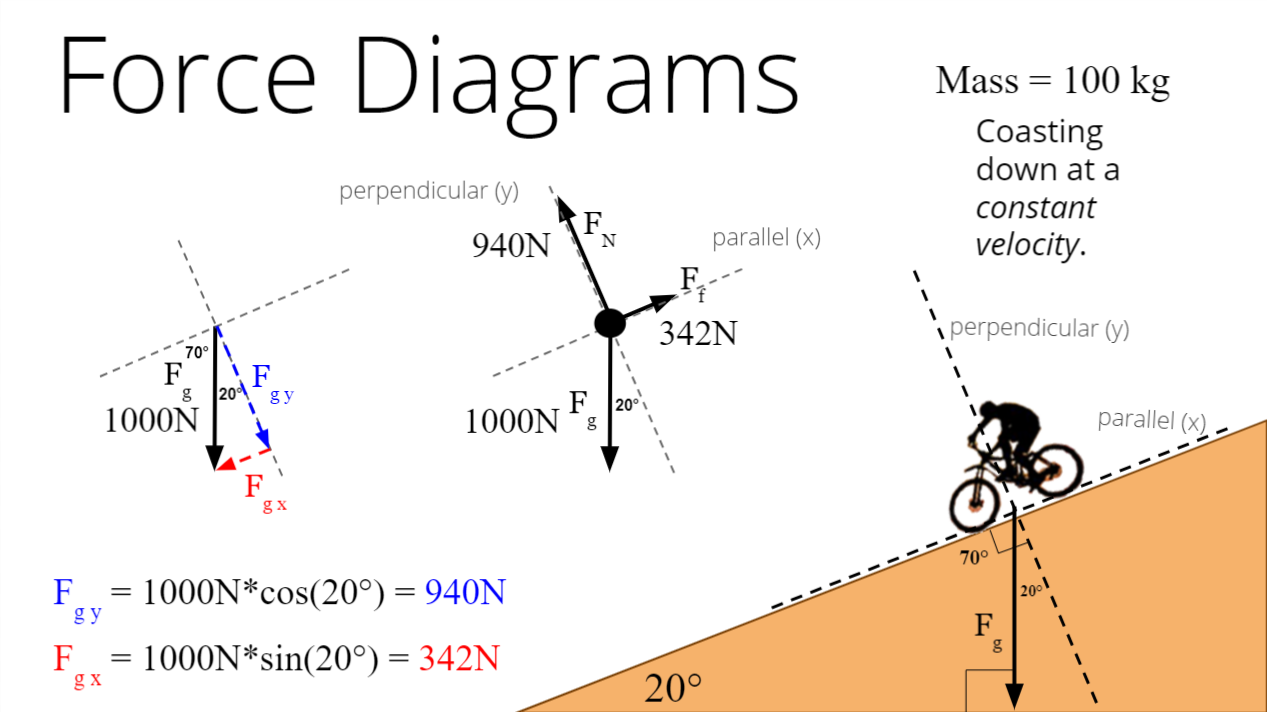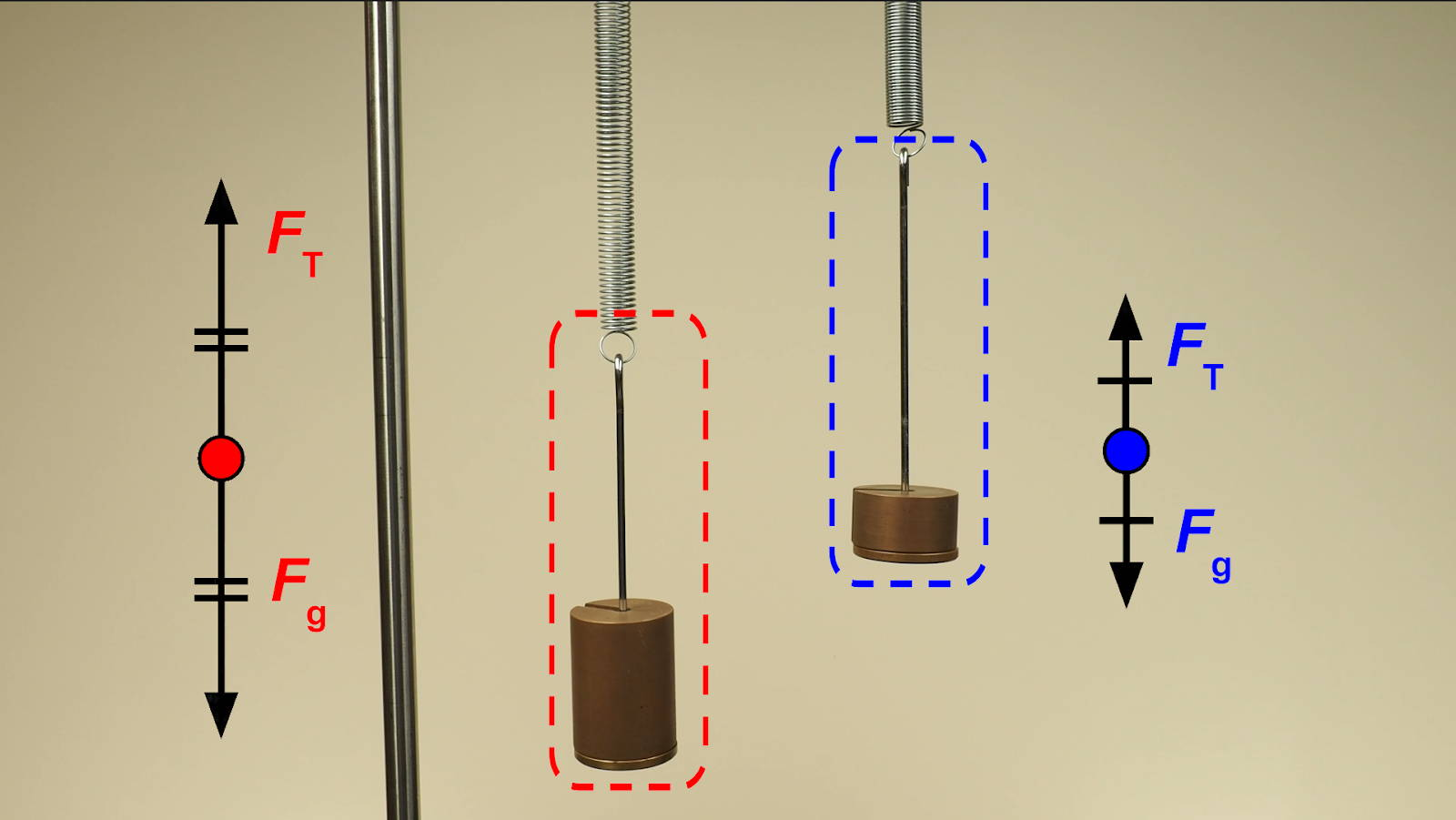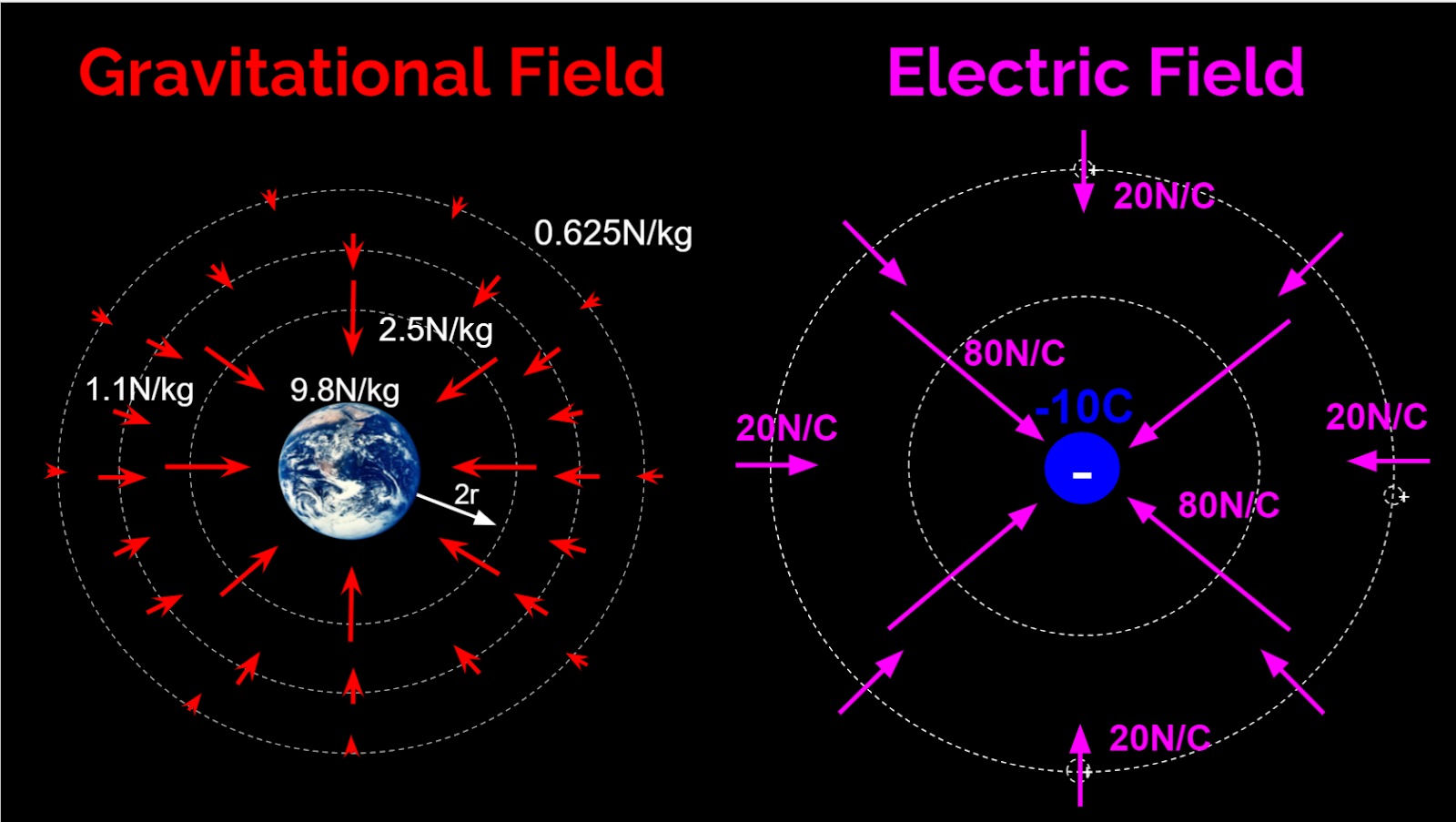# Featured Products

INTRODUCTION

Students often have a hard time distinguishing between the concepts of mass and weight. Often in their minds, the words are interchangeable.

Walk down any grocery store aisle and this confusion is only reinforced by the labels included with food products. The packaging has units of grams and ounces next to one another. Doesn’t this mean these numbers are measuring the same thing? Measuring your own weight or the weight of a package to ship involves a scale or electronic balance. These measuring devices will typically also have options to display both units of mass and weight.The following article describes an introductory physics lab that helps students separate the concepts of mass and weight, and uses experimental data to build a model of the relationship between the two variables. This lab incorporates the modeling method of instruction, which involves developing a procedure, data collection, data analysis, and collaborative discussion. The goal is to develop a model which describes the relationship between two variables, specifically how gravitational acceleration and mass determines weight. This type of lab activity allows students to experience the process of science while also building a correct scientific understanding of the world around them.

The following equipment is needed for each lab group.

PRE-LAB DISCUSSION

At the start of the year in physics, students need to learn how to construct both qualitative and quantitative force diagrams or “free-body diagrams”. Understanding the relationship between mass and weight is an important skill in physics for students to learn. Creating quantitative force diagrams often starts with calculating the size of the gravitational force given an object’s mass. For example, if an object of known mass is moving at constant speed on an incline, students need to be able to calculate both the size of the frictional force and the normal force.During the pre-lab discussion, you want to present the students with a scenario that helps them link the concepts of mass and weight, and get them thinking about how the two variables are related. Start by hanging two different masses, side by side, using the same strength springs. Choose a large enough mass so one of the objects noticeably stretches the spring farther when hanging at rest.

While you hang each object, tell the students to draw a qualitative force diagram for each of the objects. Remind them to carefully choose the length of each included force arrow to indicate if any of the forces are larger than the others, or if two forces are the same size. Most students will draw a tension force pointing up that is the same size as the gravitational force for each of the hanging objects. Several students will also draw the gravitational force and tension force larger for the object whose supporting spring stretched the farthest.Ask volunteers to explain why they included the specific forces for each object and the reason for the length of each included force. Through a guided conversation, the class should conclude that the spring with the greatest stretch is exerting the largest tension force on the hanging mass; and since the hanging object is at rest, the gravitational force is equal in size to this larger tension force. Even though we can’t directly see the size of the gravitational forces on each of the hanging objects, we can conclude that one of the objects is experiencing a larger gravitational force than the other object. Remind the students that the word “weight” refers to the size of the gravitational force experienced by an object.

Ask the students what they think causes one of the objects to have a larger weight or feel a larger gravitational force of attraction than the other. A conversation about the cause of the difference in weight usually involves a discussion about each object’s size, volume, and amount of matter or mass. Through discussion, the students should realize that an object’s size or volume must NOT be the cause of the difference in weight, since the weights of large volume objects are not always bigger than the weights of smaller volume objects. You can guide the class discussion so students recognize that one of the hanging objects has a larger weight or feels a larger gravitational force because it contains more matter or has a larger mass. Write the definition of mass on the board: mass is a quantitative measure of the amount of matter in an object.

Now that the students see there is some kind of relationship between an object’s mass and its weight, you can introduce the lab investigation. Tell students that they are going to do an investigation to determine the relationship between the force of gravity on an object and its mass. Write this purpose statement on the board.

Purpose Statement: To determine the relationship between the force of gravity on an object and its mass.

DATA COLLECTION

This is where you can help guide students through a procedure for collecting the needed data. To determine the relationship between the force of gravity on an object and its mass, the students will need to collect a variety of different mass and gravitational force measurements. Most students will have experience in measuring mass using an electronic balance or triple beam balance but will need guidance measuring the size of the gravitational force.

Bring the students’ attention back to the force diagrams that were previously discussed. As long as the hanging object is at rest, the gravitational force will be equal in size to the supporting tension force. If the students can quantify the tension force, they will also have the size of the gravitational force. Remind students that the farther a spring is stretched, the more force it exerts, pulling back against the object stretching it. A spring scale is a device that uses this concept to measure the amount of tension in the spring based on how far it is stretched. If the masses are hung from a spring scale, students are now able to measure both the size of tension and the force of gravity.

In my classroom, this is the first time students have measured the size of any force, so I use this data collection discussion to introduce “newtons”: the metric unit used to measure the size of forces. This is where you can also introduce force sensors: a “high-tech” way to measure the size of a force. You can tell students that force sensors allow more precise force measurements. Force sensors convert a mechanical force input into an electrical output. When properly calibrated, this electrical output allows the sensor to display the size of the force input.

Make sure students collect a wide range of at least 6 to 8 different values for the mass and gravitational force. Forces should be measured in units of newtons and masses should be measured in units of kilograms. Also, remind students that the recorded masses must include the mass of the hanger used in the experiment.

DATA ANALYSIS

To analyze the collected mass and gravitational force data, have the students graph their data by placing the gravitational force values on the y-axis and the mass values on the x-axis. Show students how they can write an equation from their graph showing the algebraic relationship between the force of gravity and the mass.

Any linear relationship can be written in the form of y = mx+b, but you want your students’ equations to include the specific variables and values from the graph of their specific data. The following steps can be used to guide students in writing their linear equations from their graphed data. When students first learn to write an equation from a linear fit, it is helpful for them to write out each of the three steps.

Step 1: Write the general slope-intercept form of a line: y=(m)x+b
Step 2: Replace “y” and “x” in the general equation with symbols that represent the variables graphed on each axis. In this lab the letter “m” is used to represent the mass, and “Fg” is used to represent the size of the gravitational force.
Step 3: Replace “m” and “b” with the numerical values and units of measure for the graph’s slope and y-intercept found using a linear fit of the graph.

Example: The slope of a student’s linear graph is +10N/kg and the y-intercept is -0.0252N

Step 1: y = (m)x + b
Step 2: Fg = (m)m + b
Step 3: Fg = (10N/kg)m - 0.0252N

Ask each lab group to discuss the shape of their graph and the significance or meaning of both the slope and y-intercept of their graph and equation. These questions will be the main focus of the collaborative discussion when students share their results with the rest of the class.

CONCLUSION DISCUSSION

To facilitate a whole-class conversation about the relationship between the force of gravity on an object and its mass, have each lab group record their graph and resulting equation on a large whiteboard. Have the class circle up so that everyone can clearly see the graphs and equations on each whiteboard. Remember that your goal is to help facilitate a conversation that allows your students to make connections and draw conclusions from the graphs and equations.

Start by asking students to compare the graphs and equations on the whiteboards and identify any similarities or differences they see. For this lab, students will have similar graph shapes, slope values, and y-intercept values. Once the similarities and differences are identified, the rest of the conclusion discussion should focus on what the shape of the graph suggests about the relationship between variables, the meaning of the slope, and the significance of the y-intercept.

After the students identify the shape of all the graphs is linear, ask them what this indicates about the relationship between the force of gravity on an object and its mass. The students should be able to identify that the linear shape suggests that the force of gravity will increase in size by the same amount when the mass is increased in equal increments. When looking at the slope values it should be clear that the force of gravity in all cases seems to increase by approximately 10N for each 1kg of mass. This is the meaning of the slope you want students to recognize: that there is approximately 10N of gravitational pull on every kilogram of an object’s mass.

When the students look at the values of the y-intercepts they will have small positive or negative values. The question becomes whether these values are significant or insignificant. To help students judge the significance of a y-intercept, it is not enough to just look at the value. The value of the y-intercept must be compared with the range of values collected on the y-axis, in this case, the gravitational force values. The threshold is somewhat arbitrary, but I tell my students that the y-intercept is insignificant if it is less than 5% of the maximum y-value. If this is the case, the y-intercept of the linear fit could reasonably be explained as a result of measurement errors. The y-intercept can also be considered insignificant if it can be reasoned away. Have the students think about what they would expect the y-intercept to be in this experiment. Here is a way you could ask the question: “As the mass of the hanging object gets smaller and smaller, approaching zero, what would you expect the value of the gravitational force to approach? Students will be able to identify that as the mass of the object approaches zero, they would expect the force of gravity on that object to also approach zero. If the y-intercept can be reasoned away, it can be left out of the algebraic equation.

After the students reach a consensus about the meaning of the slope and the significance of the y-intercept, you can introduce the concept of “gravitational field strength” and write the general equation on the board. The general equation shows the relationship between the force of gravity on an object and the object’s mass. Tell students that the formal name given to the slope in this experiment is the Earth’s “gravitational field strength”, the size of the gravitational force experienced by each kilogram of an object’s mass near the Earth’s surface. The accepted gravitational field strength for the Earth is 9.8 newtons for each kilogram. The symbol used to represent gravitational field strength is a lower case “g”, so the general equation can be written as the force of gravity exerted on an object is equal to the gravitational field strength of the planet multiplied by the object’s mass. The equation is usually written as Fg = mg.

After this lab experience, your students should have a much clearer understanding of the meaning of both mass and weight and a quantitative way to think about how the two are related.

EXTENSION

As an extension, ask the students how they would expect the collected data to change if the experiment was repeated on the earth’s moon. Ask students if the shape of the graph, the slope, or the y-intercept would change and why. Most students understand that they weigh less on the moon’s surface compared with the earth’s surface, but they will need to think through how this would affect the results of the experiment performed on the moon. Students should identify that the shape of the graph and the y-intercept would remain unchanged, but the graph’s slope would be smaller.

Since an object’s mass is a measure of the amount of matter in an object, this value will stay the same regardless of the object’s location in space. Each kilogram of an object’s mass will feel different amounts of gravitational pull from the planet or moon it is near. Tell students that if an object is on the moon’s surface, each kilogram of its mass will feel a gravitational attraction of about 1.67 newtons of force. This is the moon’s surface gravitational field strength: 1.67 N/kg. The moon’s surface gravitational field strength is approximately one-sixth the size of the earth’s surface gravitational field strength.

Later, the concept of gravitational field strength will be helpful when students are introduced to the concept of electric field strength. There are many parallels between the gravitational field produced by a planet and the electric field produced by a negative electric charge.An object with mass will produce a gravitational field. The gravitational field will point in the direction a positive “test mass” would feel a gravitational force, and the gravitational field strength at that location indicates the size of the gravitational force for each unit of mass of the “test mass”. The gravitational field strength has units of newtons for each kilogram (N/kg). An object with a negative electric charge will produce an electric field. The electric field will point in the direction a positive “test charge” would feel an electric force, and the electric field strength indicates the size of the electric force for each unit of charge of the “test charge”. The electric field strength has units of newtons for each coulomb (N/C).

If you are interested in learning more about these types of guided inquiry labs used in the modeling method of instruction visit www.modelinginstruction.org.

Happy investigating!

Aaron Debbink

# Featured ProductsAaron Debbink
Physics Instructor
Indian Hill High School
Cincinnati, OH

Aaron Debbink is a physics teacher with 16 years of classroom experience who has an undergraduate engineering degree and a masters degree in physics. He is passionate about building a classroom culture that values exploration and a drive for understanding. Aaron uses Modeling Instruction in his introductory and AP physics classes and has been a Modeling Instruction workshop leader since 2011. Aaron is a Knowles Teaching Fellow and the recipient of a 2019 Yale Educator Award.

September 10, 2021 Aaron Debbink